Courses

# RD Sharma Solutions (Part - 1) - Ex-13.1, Simple Interest, Class 7, Math Class 7 Notes | EduRev

## Class 7: RD Sharma Solutions (Part - 1) - Ex-13.1, Simple Interest, Class 7, Math Class 7 Notes | EduRev

The document RD Sharma Solutions (Part - 1) - Ex-13.1, Simple Interest, Class 7, Math Class 7 Notes | EduRev is a part of the Class 7 Course RD Sharma Solutions for Class 7 Mathematics.
All you need of Class 7 at this link: Class 7

#### Question 1:

Find the simple interest, when:
(i) Principal = Rs 2000, Rate of Interest = 5% per annum and Time = 5 years.
(ii) Principal = Rs 500, Rate of Interest = 12.5% per annum and Time = 4 years.
(iii) Principal = Rs 4500, Rate of Interest = 4% per annum and Time =  months.
(iv) Principal = Rs 12000, Rate of Interest = 18% per annum and Time = 4 months.
(v) Principal = Rs 1000, Rate of Interest = 10% per annum and Time = 73 days.

(i) Principal (P) = Rs 2000
Rate of interest (R) = 5% p.a.
Time (T) = 5 years

Simple interest =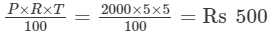(ii)  Principal (P) = Rs 500
Rate of interest (R) = 12.5% p.a.
Time (T) = 4 years
Simple interest =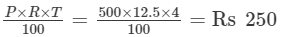(iii) Principal (P) = Rs 4500
Rate of interest (R) = 4% p.a.
Time (T) = 6 months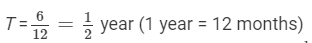Simple interest =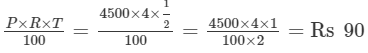(iv) Principal (P) = Rs 12000
Rate of interest (R) = 18% p.a.
Time (T) = 4 months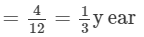(1 year = 12 months)

Simple interest =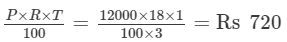(v) Principal (P) = Rs 1000
Rate of interest (R) = 10% p.a.
Time (T) = 73 days =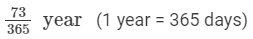Simple interest =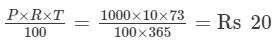#### Question 2:

Find the interest on Rs 500 for a period of 4 years at the rate of 8% per annum. Also, find the amount to be paid at the end of the period.

Principal amount (P) = Rs 500
Time period (T) = 4 years
Rate of interest (R)  = 8% p.a.

Interest =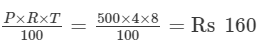Total amount paid = Principal amount + Interest = Rs 500 + 160
= Rs 660

#### Question 3:

A sum of Rs 400 is lent at the rate of 5% per annum. Find the interest at the end of 2 years.

Principal amount (P) = Rs 400
Time period (T) = 2 years
Rate of interest (R)  = 5% p.a.

Interest paid after 2 years=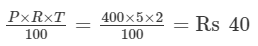#### Question 4:

A sum of Rs 400 is lent for 3 years at the rate of 6% per annum. Find the interest.

Principal amount (P) = Rs 400
Time period (T) = 3 years
Rate of interest (R)  = 6% p.a.
Interest after 3 years =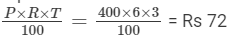#### Question 5:

A person deposits Rs 25000 in a firm who pays an interest at the rate of 20% per annum. Calculate the income he gets from it annually.

Principal amount (P) = Rs 25000
Time period (T) = 1 year
Rate of interest (R)  = 20% p.a.
Annual interest =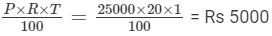#### Question 6:

A man borrowed Rs 8000 from a bank at 8% per annum. Find the amount he has to pay after 412412years.

Principal amount (P) = Rs 8000
Time period (T) =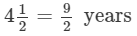Rate of interest (R)  = 8% p.a.
Interest =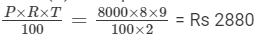Total amount paid after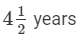= Principal amount + Interest = Rs 8000 + Rs 2880  = Rs 10880

#### Question 7:

Rakesh lent out Rs 8000 for 5 years at 15% per annum and borrowed Rs 6000 for 3 years at 12% per annum. How much did he gain or lose?

Principal amount lent out by Rakesh (P) = Rs 8000
Time period (T) = 5 years
Rate of interest (R)  = 15% p.a.
Interest =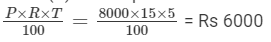Principal amount borrowed by Rakesh (P) = Rs 6000
Time period (T) = 3 years
Rate of interest (R) = 12% p.a.
Interest =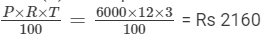Amount gained by Rakesh = Rs 6000 − Rs 2160 = Rs 3840

#### Question 8:

Anita deposits Rs 1000 in a savings bank account. The bank pays interest at the rate of 5% per annum. What amount can Anita get after one year?

Principal amount (P) = Rs 1000
Time period (T) = 1 year
Rate of interest (R) = 5% p.a.

Interest =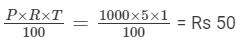Total amount paid after 1 year = Principal amount + Interest = Rs 1000 + Rs 50  = Rs 1050

#### Question 9:

Nalini borrowed Rs 550 from her friend at 8% per annum. She returned the amount after 6 months. How much did she pay?

Principal amount (P) = Rs 550
Time period (T)  = 6 months =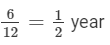(1 year = 12 months)

Interest =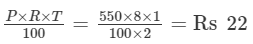Total amount paid after 6 months = Principal amount + Interest = Rs 550 + Rs 22 = Rs 572

#### Question 10:

Rohit borowed Rs 600000 from a bank at 9% per annum for 2 years. He lent this sum of money to Rohan at 10% per annum for 2 years. How much did Rohit earn from this transaction?

Principal amount lent out by Rohit (P) = Rs. 60000
Time period (T)        = 2 years
Rate of interest (R)  = 10% p.a.

Interest =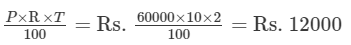Principal amount borrowed by Rohit from the bank (P) = Rs. 60000
Time period (T)                                         = 2 years
Rate of interest (R)                                   = 9% p.a.

Interest =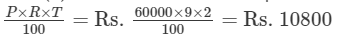Amount gained by Rohit = Rs. 12000 - 10800 = Rs. 1200

#### Question 11:

Romesh borrowed Rs 2000 at 2% per annum and Rs 1000 at 5% per annum. He cleared his debt after 2 years by giving Rs 2800 and a watch. What is the cost of the watch?

Principal amount borrowed by Romesh (P) = Rs. 2000
Time period (T)        = 2 years
Rate of interest (R)  = 2% p.a.

Interest =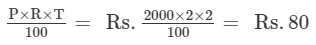Principal amount borrowed by Romesh (P) = Rs. 1000
Time period (T)        = 2 years
Rate of interest (R)  = 5% p.a.

Interest =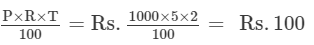Total amount that he will have to return  = Rs. 2000 + 1000 + 80 + 100 = Rs. 3180

Amount repaid = Rs. 2800
Value of the watch = Rs. 3180 - 2800 = Rs. 380

The document RD Sharma Solutions (Part - 1) - Ex-13.1, Simple Interest, Class 7, Math Class 7 Notes | EduRev is a part of the Class 7 Course RD Sharma Solutions for Class 7 Mathematics.
All you need of Class 7 at this link: Class 7Use Code STAYHOME200 and get INR 200 additional OFF Use Coupon Code
All Tests, Videos & Notes of Class 7: Class 7

### Up next### Top Courses for Class 7## RD Sharma Solutions for Class 7 Mathematics

97 docs

### Up next### Top Courses for Class 7Track your progress, build streaks, highlight & save important lessons and more!

,

,

,

,

,

,

,

,

,

,

,

,

,

,

,

,

,

,

,

,

,

,

,

,

,

,

,

,

,

,

;